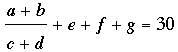SEARCH HOMEMath Central Quandaries & QueriesQuestion from mike, a student: Hello there, Problem: find 7 odd numbers who sum is equal to 30. Hint*My professor said it's possible to add 7 odd numbers and get 30 for an answer! and he said we can use Negative and rational numbers but no decimal or fractions. So is there anyway of solving this? Please get back to ASAP! thanks.Hello Mike,
Let’s try to determine if this is possible.
We know that when we add two odd numbers together, we get an even number; and when we add two even numbers, we get an even number.
So, (odd+odd)+ (odd+odd)+ (odd+odd)+odd = even+even+even+odd = odd
But we need the addition to be even, and equal to 30.  So we expect this to be impossible.

More concretely, an odd number  ‘n’ has the form, 2k + 1 where k is an integer. (Your professor said that the numbers you could use were odd integers, in other words integers which are not divisible by two)
So what you wish to show is,

(2m1 + 1) + (2m2 + 1) + (2m3 + 1) + ··· + (2m7 + 1) = 30

Where m1, m2, m3, ···, m7 are some integers.

But,

(2m1 + 1) + (2m2 + 1) + (2m3 + 1) + ··· + (2m7 + 1) = 30
2(m1 + m2 + m3 + ··· + m7) + 7 = 30
2(m1 + m2 + m3 + ··· + m7) = 23

Which is impossible, since the left hand side is divisible by two hence it is even, no matter what value is in the brackets (given that it must be an integer); and 23 is clearly not an even number.
Tyler

Mike,

Tyler is correct, adding an odd number of odd integers gives an odd integer. I wondered however about your professor saying you could use rational numbers so are you allowed to do something like?

If this is allowed can you find odd integers a, b, c, d, e, f and g so that the equation is true?

HarleyMath Central is supported by the University of Regina and The Pacific Institute for the Mathematical Sciences.